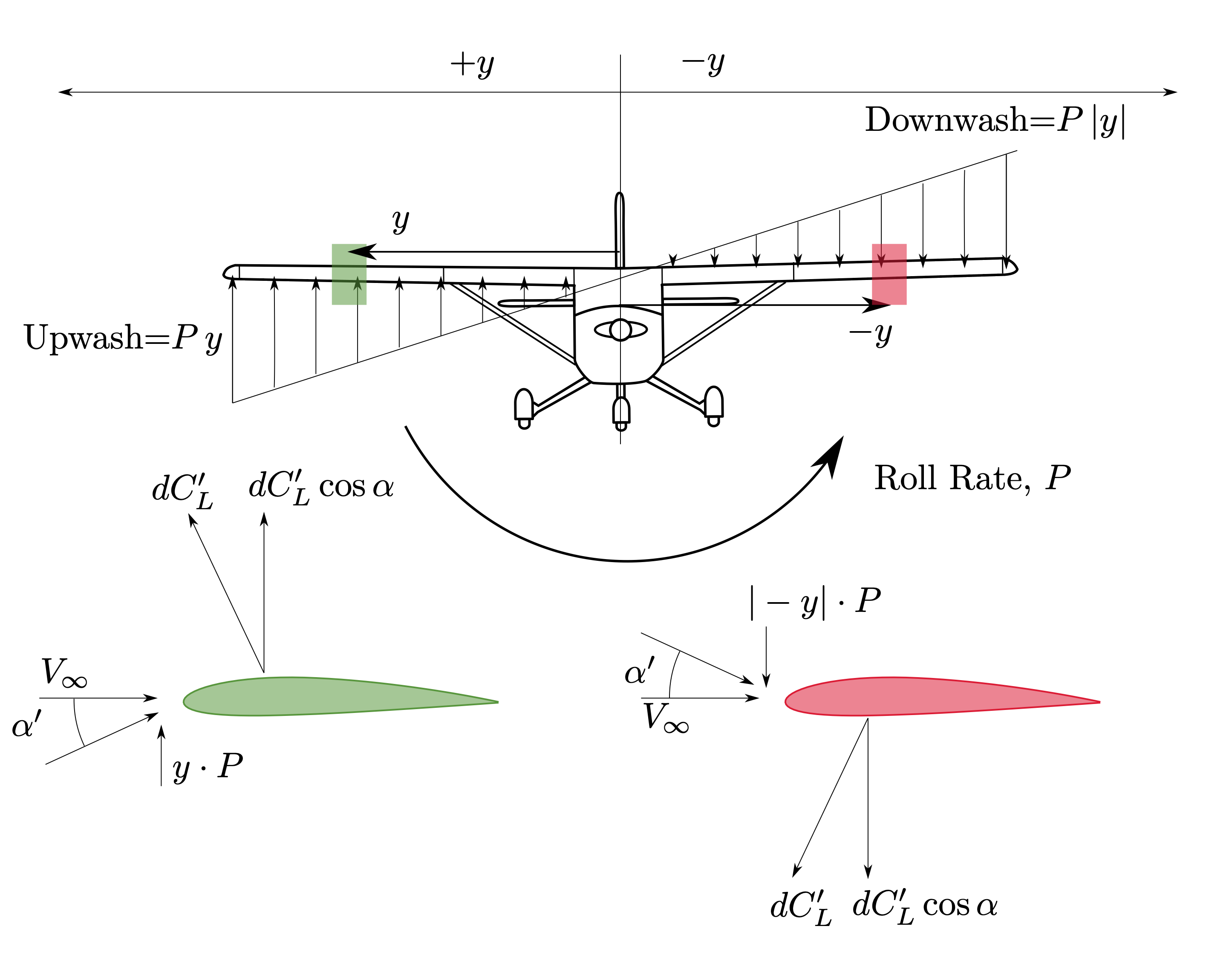# Lateral Stability#

Lateral motions refer to the aircraft response in roll. In a static sense, there is no such concept as “roll stiffness” because there is no aerodynamic roll angle (the aircraft doesn’t know if the wind vector is rotated about its own axis).

…the concept of roll stability, in the static sense, does not exist. At the most one could say that an airplane possesses neutral static stability in roll.

—Aerodynamics, Aeronautics and Flight Mechanics, page 545 :cite:MacCormick:1995wq

$C_{\ell} = C_{\ell_\beta}\cdot\beta + C_{\ell_P}\cdot P + C_{\ell_{\delta_a}}\cdot\delta_a$

Some (mostly online, but that’s what you guys will find when searching) texts refer to the derivative $$C_{\ell_\beta}$$ as roll stiffness, and I can see why, but it’s incorrect. This derivative is dihedral effect, and is an example of a cross-coupling, which will be talked about further in cross-couplings.

The second term is the roll damping - again, this is always restorative and hence negative.

## Roll damping#

The parameter $$C_{\ell_{P}}$$ or $$C_{\ell_\bar{p}}$$ is known as roll damping, and refers to the rate of change of rolling moment with roll rate. Since both $$\ell$$ and $$P$$ are defined as a right-handed rotation, the stability condition for both of these is a negative derivative.

Roll damping is always restorative - consider a positive roll rate, $$P$$, and the contribution due the aircraft wings:

• Starboard wing down $$=$$ upwash$$\,\therefore\alpha\uparrow\,\therefore C_L\uparrow\,\therefore C_\ell=f(y\cdot C_L)\downarrow$$

• Port wing up $$=$$ downwash$$\,\therefore\,\alpha\downarrow\,\therefore C_L\downarrow\,\therefore C_\ell=f(-y\cdot C_L)\downarrow$$

• Hence an increase in roll rate $$P$$ causes a decrease in rolling moment $$L$$

• A negative roll rate similarly causes an increase in rolling moment

• $$\therefore C_{\ell_P}<0$$

The contribution from the wings to roll damping is only part of the aerodynamic roll damping - similar contributions can be found from both the horizontal and vertical stabilisers, and a viscous component can be considered from the different surfaces including the fuselage.

The wing contribution is by far the largest, though, due to the greater moment arm that increases both the angle of attack change, and the moment produced by the lift increase/decrease.

### Numerical Estimates#

An estimate can be considered of the roll damping based upon the wing contribution. In a simplified model of the aerodynamics, a strip model of the wing can be utilised. Consider a section of the wing at location $$y$$, a wing element of span $$\text{d}y$$Fig. 36 Upwash and Downwash on wings due to positive roll rate#

The vertical velocity, positive in the sense of an upwash is $$P\cdot y$$.

The change in effective angle of attack, $$\alpha^\prime$$, is therefore

$\alpha^\prime=\tan^{-1}\frac{y\,P}{V_{\infty}}$

Which, subject to the small angle approximation (expand the bit of code below if you’re interested about small angles) is

$\alpha^\prime\simeq\frac{y\,P}{V_{\infty}}$
import numpy as np
import plotly.graph_objects as go
# What about the small angle assumption?

# We often use the small angle assumption in aerodynamics, but have you over looked at its validity?
# You also may not have seen the arctangent small angle assumption

angle = np.linspace(.1, 50, 1000)
sin = np.sin(rangle)
cos = np.cos(rangle)
tan = np.tan(rangle)

sin_error = (rangle - sin)/sin*100
cos_error = (1 - cos)/cos*100
tan_error = (rangle - tan)/tan*100

fig = go.Figure()

mode='lines', name='$\\theta - \\sin\\theta$'))
mode='lines', name='$1-\\cos\\theta$'))
mode='lines', name='$\\theta-\\tan\\theta$'))
xaxis_title="$\\frac{\\theta}{\circ}$",